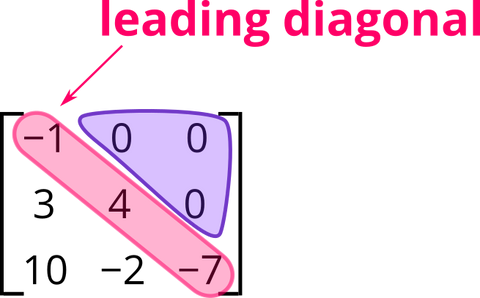# Further Mathematics - Exam Revision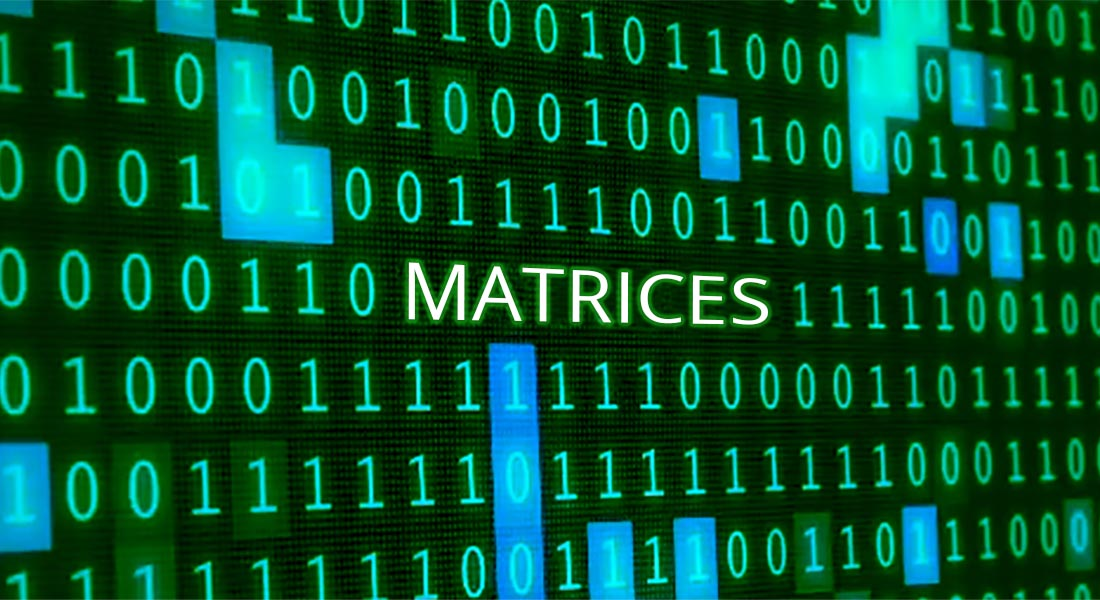## Matrices ... What are they?

Authored By Carmen Popescu-Rose 0 Comment(s)

What is a matrix?

A matrix is a useful way of representing linear relationships between variables.

Matrices look like grids or tables with lists of numbers written in rows and columns.This matrix has 2 horizontal lists of numbers called rows.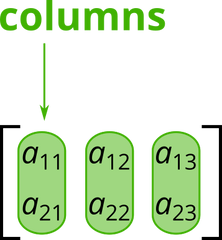This matrix has 3 vertical lists of numbers called columns.

Each element appears at the intersection between a row and a column.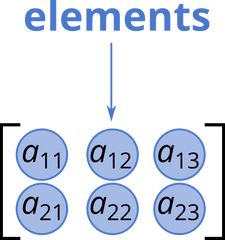This matrix has 6 elements.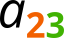This element is in row 2 and column 3.

Converting a two-way table into a matrix

The data in the table below shows that, in a group of 50 children, 5 girls have cats as pets, 17 girls have dogs as pets, 3 boys have cats as pets and 25 boys have dogs as pets.

 Pets ... and here is its corresponding matrix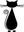Gender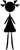5 173 25

Types of matrices

 Row matrices * one row onlyColumn matrices * one column only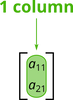Square matrices * number of rows = number of columnsIdentity matrices * 1s on the leading diagonal * 0s elsewhere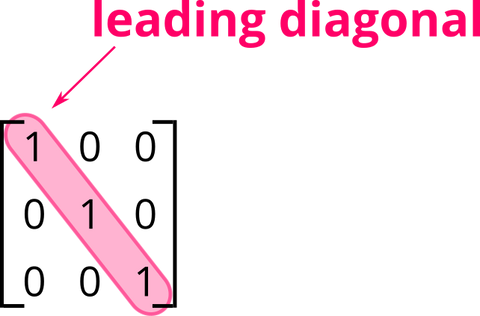Diagonal matrices * any numbers on the leading diagonal * 0s elsewhereZero matrices * all elements are 0s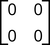Upper triangular matrices * all elements below the leading diagonal are 0sLower triangular matrices * all elements above the leading diagonal are 0s